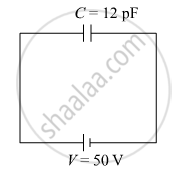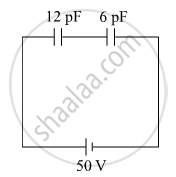PUC Karnataka Science Class 12Department of Pre-University Education, Karnataka
Share

# A 12 pF capacitor is connected to a 50 V battery. How much electrostatic energy is stored in the capacitor? If another capacitor of 6 pF is connected in series with it with the same battery connected across the combination, find the charge stored and potential difference across each capacitor. - PUC Karnataka Science Class 12 - Physics

#### Question

A 12 pF capacitor is connected to a 50 V battery. How much electrostatic energy is stored in the capacitor? If another capacitor of 6 pF is connected in series with it with the same battery connected across the combination, find the charge stored and potential difference across each capacitor.

#### SolutionE=1/2CV^2

E=1/2×12×10^-12×(50)^2

E=1.5×10^-8 JC_(eqv)=(C_1C_2)/(C_1+C_2)

=(12×6)/(12+6)

=72/18

=4×10^-12 F

Q=C_(eqv)V

=4×10^-12×50

=200×10^-12 C

Charge stored in both capacitor is 200×10^-12 C.

Voltage across 12 pF capacitor is =Q/C= (200×10^-12)/(12×10^-12)=200/12=16.67 V

Voltage across 12 pF capacitor is=Q/C= (200×10^-12)/(6×10^-12)=200/6=33.33 V

Is there an error in this question or solution?

#### APPEARS IN

Solution A 12 pF capacitor is connected to a 50 V battery. How much electrostatic energy is stored in the capacitor? If another capacitor of 6 pF is connected in series with it with the same battery connected across the combination, find the charge stored and potential difference across each capacitor. Concept: Electrostatics of Conductors.
S